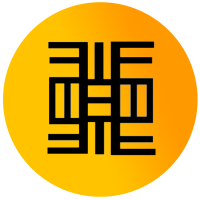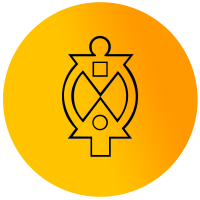# FOR HIGH SCHOOL STUDENTSDOORSTEP & ONLINE SERVICES

• New York
• NYC (New York City)
• Manhattan
• Brooklyn
• Queens
• Staten Island
• Bronx
• Long Island including the Hamptons
• Westchester
• New Jersey
• Connecticut
• and remote to any location!

CHECK US OUT

DOORSTEP & ONLINE SERVICES

• New York
• NYC (New York City)
• Manhattan
• Brooklyn
• Queens
• Staten Island
• Bronx
• Long Island including the Hamptons
• Westchester
• New Jersey
• Connecticut
• and remote to any location!

CHECK US OUTRATES

MEET OUR FOUNDER AND STAFF

THEMBA AND THE COMPETITION

FINDING A QUALIFIED TUTOR

STAFF’S BIOS

SCHOOLS WE WORK WITH

TESTIMONIALS## Algebra II

Our learning specialists and academic tutors provide 1:1 individualized tutoring in Algebra II for high school students.

Algebra II is usually the third math course in high school after students take Algebra I and Geometry. Algebra II guides students through linear equations, inequalities, graphs, matrices, polynomials and radical expressions, quadratic equations, functions, exponential and logarithmic expressions, sequences and series, probability, and trigonometry.## What’s the difference between Algebra I and Algebra II?

Algebra 1 introduces the general concepts of algebra. Students learn about variables and functions. The primary focus is solving equations. The only functions they study extensively are linear and quadratic. Students learn their basic properties, how to find their roots, how to graph them, how to convert them between different “forms,” and how to find their inverses.

Algebra II is more advanced. Students learn everything from logarithms and complex numbers to implicit functions and conics to the fundamental theorem of algebra.

## What Challenges Do Students Encounter With Algebra II?

Algebra II can be an intimidating subject for students but with the right teaching strategies, educators can engage students in the subject matter and assist them in a better understanding of the complicated concepts.

Algebra I and II are considered two of the most important pillars in mathematics. Algebra is more than just solving for x and y. It’s a way of thinking and approaching conceptual problems with variable entities and reasoning them out successfully.

Here is a list of possible challenges students might encounter with Algebra II.

Poor Mathematical Foundations

In order to solve different types of algebraic expressions, students need to first work on strengthening fundamental concepts in basic mathematics like arithmetic and number theory. They need to have a clear understanding of how basic math works in order to solve problems that contain more complex mathematical concepts. Lack of primary knowledge leads to making mistakes in the process of arriving at the conclusion.

Lack of Clarity While Solving Basic Equations

Solving equations is the center of algebra studies. Students start by learning to solve simple linear equations with just one variable and build to solve more complex and difficult problems. If students haven’t had a solid foundation in understanding the steps necessary to solve equations, it might be challenging for them. A simple way to make a student understand how to solve algebraic equations is by thinking of the problem as a balance. Each side has to remain balanced and the equal sign acts as the pivot.Dyscalculia

This is a learning disability that causes students to struggle with formulas, shapes, and number concepts. Dyscalculia makes it difficult for students to process math problems. Students with dyscalculia usually fall behind their peers in math.

No room for error

Unlike other subjects, there’s no room for error when it involves math. Students need to understand math concepts. Because of this, math can quickly become a frustrating and stressful experience for students.

It requires a lot of practice

Math isn’t something that students automatically “get.” It takes practice to understand math concepts. Without practice, students can have a tough time keeping up, and then the more advanced math concepts will be harder for them.

What Are The Best Strategies For Studying Algebra II?

Practice, practice, and more practice!

This isn’t much of a secret but many students overlook this essential advice when it comes to learning math. It requires plenty of ‘doing.’ It’s not a subject you can memorize from a textbook the night before an exam. Students need to understand the logic and process behind the problems, which comes naturally with practice. It also allows one to identify and work through common mistakes. In the end, practice helps students improve their math skills.

Finish homework

It goes without saying that homework is very important for studying math because students need to apply what they learned in class. Even if the course instructor doesn’t require handing in-home assignments, consider it part of math practice.

Take extensive notes

When students are in a lecture, they should take detailed notes. Instead of merely copying the math examples on the board, it’s also a great idea to write all explanations and tips stated by the professor. Later, when reviewing notes or doing homework, students might find class notes helpful. The notes will help solve difficult problems. Students will read and recall what the professor said about steps and solutions. Plus, math encourages active learning. Students are engaged while listening to the lecture and absorb the material instead of zoning out and trying to catch up later.

Get support and tutoring

There are many reasons students might need support and tutoring for math. It is a cumulative subject, meaning students need a strong foundation or they might fall behind. Sometimes students just need something explained in a different way to truly understand it.

Benefits of One on One Tutoring?

• An individual and unique learning experience
• One-on-one attention
• Improves attitude towards learning and school
• Encourages self-paced and self-directed learning
• Improves self-esteem and confidence
• Improves work and study habits
• Provides a positive workspace
• Encourages independence and responsibility
• Helps overcome learning obstacles
• Encourages the freedom to ask questions
• Improves social skills
• Increases the ability to manage one’s learning
• Challenges students
• Prepares students for college

Algebra 1

Learn about algebra 101, reciprocals, common core, algebra 1 expressions, equations, questions, and formulas.

Algebra 2: Solving Inequalities

Learn about algebra 2, algebra inequalities, algebraic equations, graphing, and inequalities.

Algebra Equations: Solving Equations by Inspection

Learn about equations by inspection, algebraic equations, and practice formula.

Algebra Basics: Introduction to Algebra

Learn about algebra basics and an introduction to algebra.

Algebra Problems: Solving Algebraic Expressions with Exponents

Learn how to solve algebraic expressions with exponents, linear, and word problems.At Themba Tutors, we will let you know what kind of help you need once we set up a free intake. Knowing the student’s strengths and weaknesses is vital for helping the student with the challenges they’re facing. We offer services that include academic coaching and tutoring based on the student’s needs. We also offer a hybrid model for those students who need help with content and improving their underlying study skills.RATES

MEET OUR FOUNDER AND STAFF

THEMBA AND THE COMPETITION

FINDING A QUALIFIED TUTOR

STAFF’S BIOS

SCHOOLS WE WORK WITH

TESTIMONIALSContact Themba Tutors!

Themba Algebra 2 Tutoring is customized for all grade and skills levels!

Call: (917) 382-8641, Text: (201) 831-9848

Email: info@thembatutors.com

(we respond to email right away!).